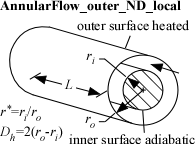Contents - Index

AnnularFlow_outer_ND_localProcedure AnnularFlow_outer_ND_local(Re, Pr, xoverD_h, r|star, relRough: Nusselt_T_x,Nusselt_H_x, f_x)  returns the lower and upper bounds on the local Nusselt number for fully-developed flow in an annulus characterized by inner and outer radii.  The inner wall of the annulus is assumed to be insulated so heat transfer occurs across the outer wall.  The area used for heat transfer should be the area of this wall.  The procedure also returns the local friction factor for these conditions.

Inputs:

Re - Reynolds number based on properties evaluated at the bulk average temperature

Pr - Prandtl number of the fluid evaluated at the bulk average temperature

xoverD_h - ratio of local axial position to hydraulic diameter.

r|star - ratio of the inner to the outer radius of the annulus   This value should be <=1 and >0

RelRough - the ratio of the dispersions on the wall of the tube to the tube diameter (must be between 0 and 0.05)

Outputs:

Nusselt_T_x - lower bound on the local Nusselt number assuming a constant outer wall temperature

Nusselt_H - upper bound on the local Nusselt number assuming a constant outer wall heat flux

f -  local friction factor [-]

Notes:

This procedure  determines local values by differentiating the average values from procedure AnnularFlow_outer_N.

Two values of the Nusselt number coefficient are returned that should provide upper and lower bounds.  These  coefficients are both for the outer annular wall with an insulated inner wall.  They both correspond to fully developed flow. For turbulent flow, these values are identical.

Example:

Re=1500

Pr=0.72

xoverD_h=20

relRough=0

call AnnularFlow_ND_local(Re,Pr,xoverD_h,0.35,RelRough: Nusselt_T_x, Nusselt_H_x, f_x)

{Solution:

f_x=0.07316

Nusselt_H_x=5.473

Nusselt_T_x=4.977

}